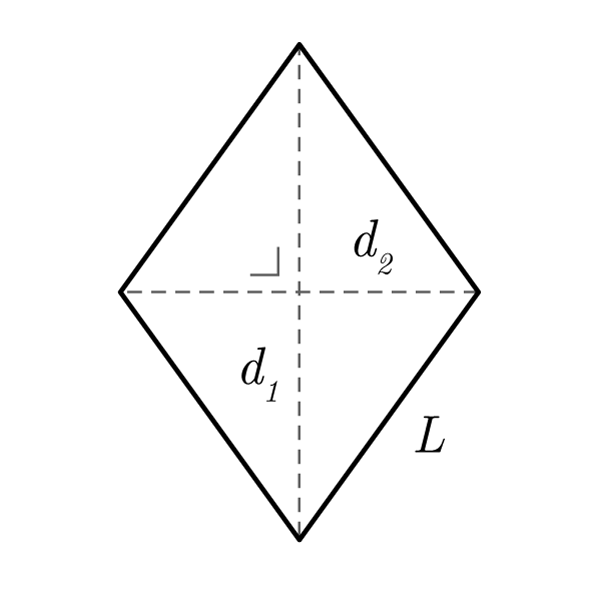# Rhombus

 Longer Diagonal $$d_{1}$$ Shorter Diagonal $$d_{2}$$ Side $$S$$ Longer Semi-Diagonal $$\frac{d_{1}}{2}$$ Shorter Semi-Diagonal $$\frac{d_{2}}{2}$$
$$2p = S \times 4$$
Perimeter
$$S = \frac{2p}{4}$$
$$A = \frac{d_{1} \times d_{2}}{2}$$
Area
$$d_{1} = \frac{2A}{d_{2}}$$
Longer Diagonal
$$d_{2} = \frac{2A}{d_{1}}$$
Shorter Diagonal
$$S = \sqrt{ {\left(\frac{d_{1}}{2}\right)}^{2} + {\left(\frac{d_{2}}{2}\right)}^{2} }$$
Side (Pythagoras' theorem)
$$\frac{d_{1}}{2} = \sqrt{ {S}^{2} - {\left(\frac{d_{2}}{2}\right)}^{2} }$$
Longer Semi-Diagonal
$$\frac{d_{2}}{2} = \sqrt{ {S}^{2} - {\left(\frac{d_{1}}{2}\right)}^{2} }$$
Shorter Semi-Diagonal

## Definition

A rhombus is a quadrilater with all sides congruent.

## Properties

1. Four congruent sides, opposite sides are parallel
2. Opposite angles are congruent, consecutive angles are supplementary (their sum is 180°)
3. Diagonals are perpendicular
4. Diagonals meet at a point called rhombus' center. The center divides the diagonals into two equal semi-diagonals
5. Diagonals makes four congruent right triangles, in which the hypotenuse is represented by the rhombus' side, and the cathetus' by the semi-diagonals## Rhombus Formulas

Data Formula
Perimeter 2p = S× 4
Area A = (d1 × d2) / 2
Side S = 2p / 4
Side S = √[ (d1 / 2)2 + (d2 / 2)2 ]
Longer Diagonal d1 = (2 × A) / d2
Shorter Diagonal d2 = (2 × A) / d1
Longer Semi-Diagonal d1 / 2 = √[ S2 - (d2 / 2)2 ]
Shorter Semi-Diagonal d2 / 2 = √[ S2 - (d1 / 2)2 ]